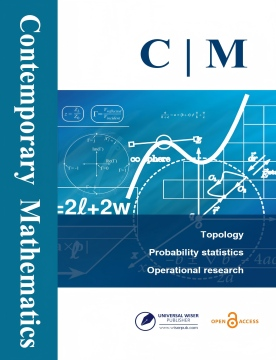# Contemporary Mathematics

ISSN: 2705-1064 (Print) 2705-1056 (Online) Description

Contemporary Mathematics is a quarterly peer-reviewed scientific journal. Our ultimate goal is to make the journal an important source for publishing high quality papers related to the development of contemporary mathematics as well as a continuing and evolving source of interesting and relevant problems for researchers.

The scope of this journal includes theoretical, numerical, and experimental studies in all fields of mathematics and the mathematical sciences. A broad spectrum of topics are covered like: mathematical theory, pure mathematics, algebra, geometry and topology, complex analysis, differential equations, industrial mathematics, computational mathematics, discrete mathematics, etc.

# Latest Articles

## Open AccessArticle

A Nullclines Approach to the Study of 2D Artificial Network

ABSTRACT: The system of two the first order ordinary differential equations arising in the gene regulatory networks theory is studied. The structure of attractors for this system is described for...

## Open AccessArticle

Approximate Solutions for Solving Fractional-order Painlevé Equations

ABSTRACT: In this work, Chebyshev orthogonal polynomials are employed as basis functions in the collocation scheme to solve the nonlinear Painlevé initial value problems known as the first and second...

## Open AccessArticle

Novel Exact Solitary Wave Solutions for the Time Fractional Generalized Hirota–Satsuma Coupled KdV Model Through the Generalized Kudryshov Method

ABSTRACT: In the current article, the generalized Kudryshov method is applied to determine exact solitary wave solutions for the time fractional generalized Hirota–Satsuma coupled KdV model. Here,...

## Open AccessArticle

Numerical Approximation of Modiﬁed Non-linear SIR Model of Computer Viruses